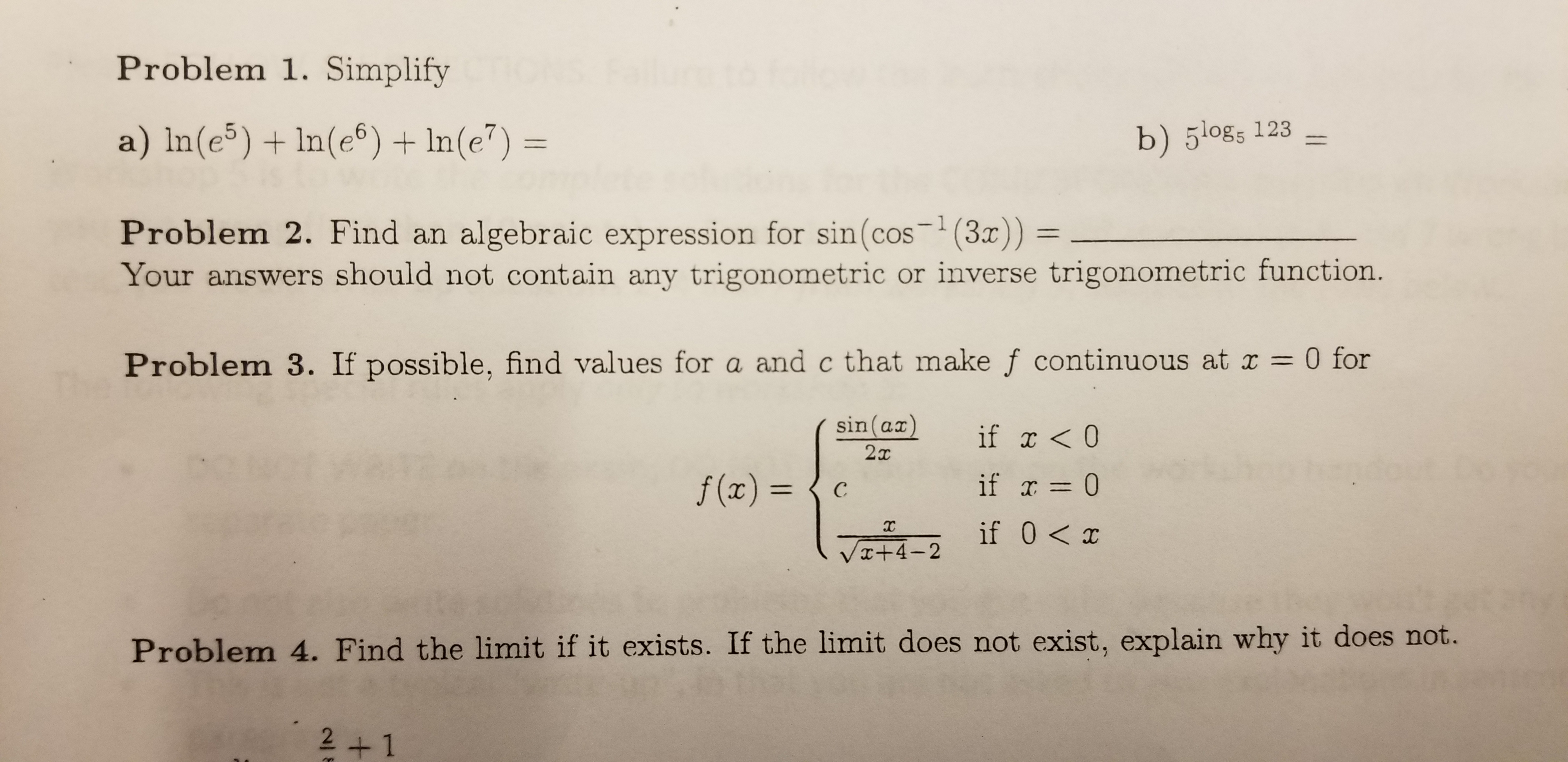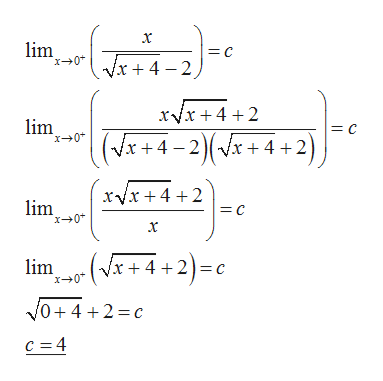# Problem 1. Simplifya) In(e5) In(e6) In(e7) =b) 5l0gs 123algebraic expression for sin(cos (3)) =Your answers should not contain any trigonometric or inverse trigonometric function.- 1Problem 2. Find an0 forProblem 3. If possible, find values for a and c that make f continuous at r1sin (ax)if x

Question
76 viewshelp_outlineImage TranscriptioncloseProblem 1. Simplify a) In(e5) In(e6) In(e7) = b) 5l0gs 123 algebraic expression for sin(cos (3)) = Your answers should not contain any trigonometric or inverse trigonometric function. - 1 Problem 2. Find an 0 for Problem 3. If possible, find values for a and c that make f continuous at r 1 sin (ax) if x<0 2т if 0 f(x) = с if 0 x VI+4-2 Problem 4. Find the limit if it exists. If the limit does not exist, explain why it does not. 2+1 fullscreen
check_circle

Step 1

Given:

Step 2

The function is continuous at x = 0 only when,

Step 3

Therefore...help_outlineImage Transcriptioncloselim = C x0 Vx 4 2 xVx+4 2 lim x0 = C (Vx+4-2(x+4+ 2) xvx+4+2 lim = C x+0 x -(x+4+2)=c lim C x0 VO42 c c =4 fullscreen

### Want to see the full answer?

See Solution

#### Want to see this answer and more?

Solutions are written by subject experts who are available 24/7. Questions are typically answered within 1 hour.*

See Solution
*Response times may vary by subject and question.
Tagged in

### Continuity